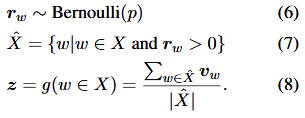# Implementing Word Dropout

Is there a simple way to implement word dropout as described in “Deep Unordered Composition Rivals Syntactic Methods for Text Classification” (http://aclweb.org/anthology/P15-1162)? The approach is described below.I have attempted the following:

``````import torch
from torch import nn
from torch.distribitutions.bernoulli import Bernoulli

nwords, dim = 4, 3
emb = nn.Embedding(nwords, hidden)
input = torch.LongTensor([[0, 1, 2, 2, 1],
[0, 3, 2, 1, 2]])
out = emb(input)
rw = Bernoulli(0.3).sample((nwords, ))
``````

But I’m stuck here. What I would like to do is something like `out.where(indicies(rw)).mean(dim=1)` but that’s not quite right.

``````import torch
from torch import nn
from torch.distribitutions.bernoulli import Bernoulli

nwords, dim = 4, 3
emb = nn.Embedding(nwords, dim)
input = torch.LongTensor([[0, 1, 2, 2, 1],
[0, 3, 2, 1, 2]])

out = emb(input)
rw = Bernoulli(0.3).sample((out.shape, ))

out[:, rw==1].mean(dim=1)
``````
1 Like

Thanks - that works.